[Mathematics] [Other] [Home]

# The Fourth Dimension

The five basic Platonic solids, the tetrahedron, cube, octahedron, dodecahedron, and icosahedron, are illustrated in the diagram below.Below each one is what is called a Schäfli symbol. This is a way of describing not only regular polyhedra in three dimensions, but also regular polychora in four dimensions, or regular polytopes in any number of dimensions.

The first number indicates the number of sides in the polygon we start with.

The second indicates how many of those polygons meet at their corners to build a polyhedron.

In higher dimensions, the third number then indicates how many polyhedra meet at their edges to build the four-dimensional equivalent of a polyhedron, a polychoron. And the next number indicates how many polychora meet at a two-dimensional face to construct a five-dimensional polytope, and so on.

Since the first number is distinct from the rest, I have adopted the form [(5) 3, 3] for the Schäfli symbol usually written {5, 3, 3} (which, of course, looks like a set, and can therefore cause confusion). In the diagrams, I have gone further, putting the first number in a circle, and succeeding numbers in square cells.The diagram above illustrates the tesseract, or hypercube, the four-dimensional analogue of a cube, with the Schäfli symbol [(4) 3, 3].

The cross-like shape illustrates what the surface of a hypercube can be made from by folding, in the same way that a cross-like arrangement of six squares can be folded up to make the surface of a cube.

And the other drawing in the picture is a projection, on a flat drawing, of a projection of the skeleton of a hypercube in three dimensions.

One thing not obvious from the projection is where is the four-dimensional space that the surface of a hypercube, consisting of cubes, is enclosing. All that is visible in the projection are eight cubes; one in our space, one displaced in a fourth-dimensional direction, and six cubes connecting corresponding faces of those two cubes.

But in a three-dimensional space not displaced from our plane quite as far as the second cube mentioned is, those six cubes only intersect that space partly; as six squares, which together enclose a hollow cube. And the three-dimensional space inside those cubes, over all the three dimensional spaces extending between the solid cubes mentioned first and second, makes up the four-dimensional space inside the hypercube.

The hypercube is not the only regular polychoron. There are five others, for a total of six.

The regular polychora are:

• The pentatope, with Schäfli symbol [(3) 3, 3]. It consists of a tetrahedron, plus the point in the center of that tetrahedron, displaced in the direction of the fourth dimension, and four other tetrahedra which join each face of the first tetrahedron to that point.
• The tesseract, or hypercube, with Schäfli symbol [(4) 3, 3], which we have already met.
• The cross polychoron, with Schäfli symbol [(3) 3, 4], which is the dual of the tesseract, in the same sense that the octahedron is the dual of the cube; one can be constructed using the points in the center of each cubical "face" of the hypercube. Another construction of the cross polychoron is to consider it as consisting of a tetrahedron in our space, a tetrahedron turned upside down and rotated sixty degrees, so as to make it the dual of the first tetrahedron, displaced by a fourth-dimensional distance, and eight tetrahedra to join the four faces of each of the first two tetrahedra to the corresponding corner of the other one.
• The 24-cell, with Schäfli symbol [(3) 4, 3]. This shape is composed of 24 octahedra, and like the pentatope, is its own dual.
• The 120-cell, with Schäfli symbol [(5) 3, 3]. This shape is built up of dodecahedra. If you place twelve dodecahedra on the faces of another one, the faces adjacent to the face touching that one dodecahedron will almost touch, with a narrow wedge of space between them. Folding the twelve dodecahedra into the fourth dimension enough to make them touch produces a shallow curve, which leads to a fourth-dimensional solid with 120 dodecahedra as its faces. Besides being built from dodecahedra, it is considered the four-dimensional analogue of the dodecahedron.
• The 600-cell, with Schäfli symbol [(3) 3, 5]. This shape is built from tetrahedra, but this time five meet at each edge. It is the dual of the 120-cell.

Here is a diagram of another one of the simpler shapes among these six, the pentachoron, or pentatope, or the simplex in four dimensions: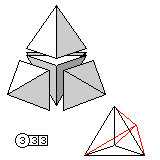and here is a diagram, this time only showing a projection, of the cross polytope in four dimensions: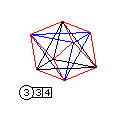In five or more dimensions, only the analogues of the tetrahedron, cube, and octahedron exist.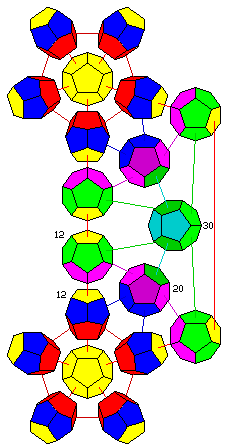There are several other sites, some of which are listed in the Links section of my page, which provide projections of the 24-cell, 120-cell, and 600-cell. These projections are, however, somewhat complicated looking, and perhaps a bit confusing. Thus, rather than making great effort to duplicate what these other pages have done, for example, this page, or this page, I have produced a diagram which may help to make sense of other representations of the 120-cell.

The diagram on the left shows a small number of the 120 dodecahedra which make up the surface of a four-dimensional 120-cell.

At the bottom, with all its faces colored yellow, is the dodecahedron that will serve as our starting point. The colors yellow and red indicate 12-fold symmetry; these faces touch other dodecahedra which, naturally enough, correspond to faces of this starting dodecahedron.

Five of the twelve dodecahedra which surround it are shown. The five faces which surround the face touching the starting dodecahedron are red. These faces are the ones with which these 12 dodecahedra touch each other.

The far face of each of these dodecahedra is also yellow, since it points in the same direction as a yellow face of the starting dodecahedron; on these faces, we build a tower of dodecahedra in a straight line which then gets bent into the fourth dimension.

The ring of five pentagonal faces on the dodecahedron which is away from the starting dodecahedron are blue. This is because they point in such a direction that the dodecahedra which these faces touch will touch three of these blue faces. And it will be situated outwards from one of the 20 corners - or vertices - of the original dodecahedron, and thus there will be 20 of these dodecahedra. (Note that, in the diagram, the number 20 is written next to one of those dodecahedra, just as the number 12 is written next to the first two dodecahedra piled on the top face of the original dodecahedron.)

The dodecahedron with three blue faces also has purple faces, with which it touches the dodecahedra above it in the main stack, and dark purple faces, by means of which these 20 dodecahedra touch each other. There is also one green face.

In the middle of the diagram vertically, with the number 30 next to it, is a dodecahedron with faces in different shades of green. This dodecahedron is situated above an edge of the original dodecahedron, so there are 30 of them, just like the diamonds on the 30-sided dice made by placing suitable pentagonal pyramids on each face of a dodecahedron.

These 30 dodecahedra are exactly halfway between the original yellow dodecahedron and the one on the opposite side of the 120-cell. It is hoped that the diagram here will help, in conjunction with other representations of this object, to illustrate how the component dodecahedra go together.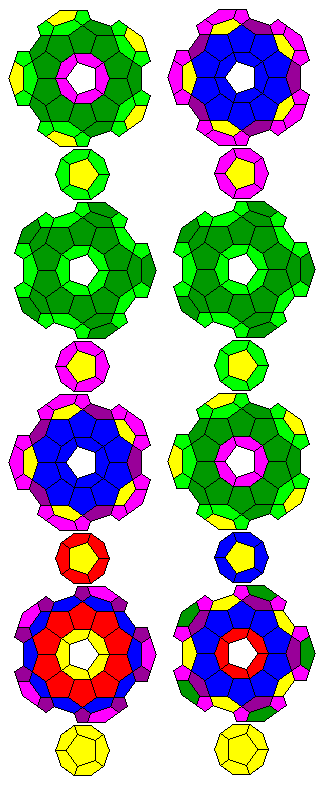However, although this diagram shows how one group of dodecahedra of each type are connected, it only hints at the symmetry involved. Thus, I have produced an additional diagram, showing layers of dodecahedra surrounding one central column of dodecahedra: in this diagram, to the right, there are two columns, the one on the left showing each layer as viewed from below, the one on the right showing each layer as viewed from above. And below is an earlier additional set of diagrams, this time showing all the dodecahedra surrounding the central all-yellow dodecahedron, a layer at a time.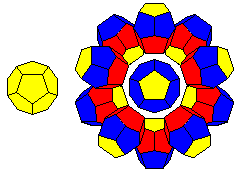This first image shows the first two diagrams in the series. The first diagram shows just the central dodecahedron by itself. The second shows it surrounded by the first twelve additional dodecahedra. (The original dodecahedron, and one of the new group of twelve, are not visible, as the diagram is an exact face-on view without perspective.) The colors are the same as in the diagram that ran down the left side of this page.This next image shows the layer of 20 dodecahedra which rest on three blue faces each of the dodecahedra in the previous layer. These dodecahedra have dark purple faces with which they touch one another, and light purple faces with which they will touch the 12 dodecahedra in the central pillars in the next layer. As well, they have dark green faces, on which the 30 dodecahedra in the last layer will rest. The 20 dodecahedra in this layer each correspond to a vertex (or corner) of the original all-yellow dodecahedron.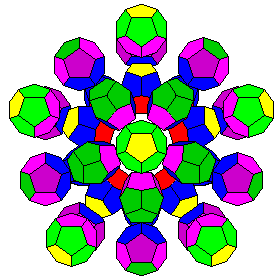This image shows another layer of 12 dodecahedra. Now, two dodecahedra are piled on top of every face of the original yellow dodecahedron. The faces of these dodecahedra, as in the previous layer of 12, which point towards and away from that original dodecahedron are yellow. Of the other 10 faces, the five near the original dodecahedron are light purple, as they rest on dodecahedra in the layer of 20 dodecahedra. The five pointing away from the original dodecahedron are light green, as they, like the dark green faces of the dodecahedra in the previous layer, will contact the 30 dodecahedra of the next layer.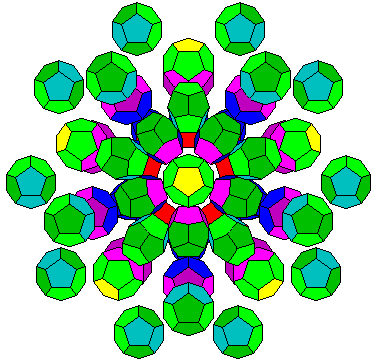Finally, we see the final layer of dodecahedra. As indicated in the diagram running along the left side of the page, this layer marks the exact midpoint of our journey from the original yellow dodecahedron. After this, the other layers will repeat in turn, until we return to a single yellow dodecahedron at the other end of the 120-cell. This layer has 30 dodecahedra in it; each one corresponds to an edge of the original yellow dodecahedron. In addition to light green and dark green faces, the dodecahedra in this layer have blue-green faces with which they contact one another.

[Mathematics] [Other] [Home]